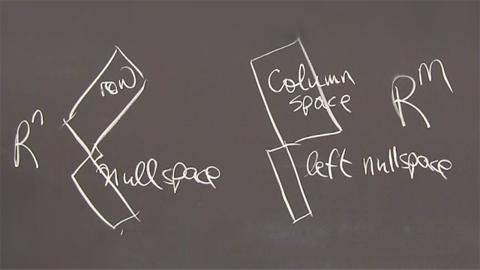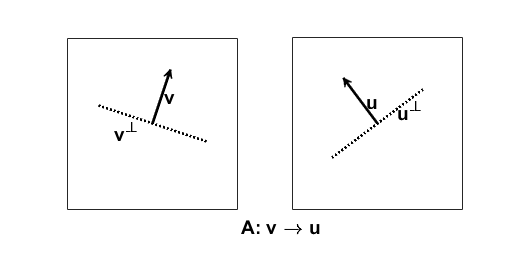# Four Fundamental Subspaces of Linear Algebra, Corrected

(Please replace the erroneous posting from yesterday, Nov. 28, with this corrected version.)

Here is a very short course in Linear Algebra. The Singular Value Decomposition provides a natural basis for Gil Strang's Four Fundamental Subspaces.Screen shot from Gil Strang MIT/MathWorks video lecture, "The Big Picture of Linear Algebra".

### Contents

#### Gil Strang

Gil Strang tells me that he began to think about linear algebra in terms of four fundamental subspaces in the 1970's when he wrote the first edition of his textbook, Introduction to Linear Algebra. The fifth edition, which was published last May, features the spaces on the cover.

The concept is a centerpiece in his video lectures for MIT course 18.06. It even found its way into the new video series about ordinary differential equations that he and I made for MIT and MathWorks. His paper included in the notes for 18.06 is referenced below.

#### The Four Subspaces

Suppose that $A$ is a $m$ -by- $n$ matrix that maps vectors in $R^n$ to vectors in $R^m$. The four fundamental subspaces associated with $A$, two in $R^n$ and two in $R^m$, are:

• column space of $A$, the set of all $y$ in $R^m$ resulting from $y = Ax$,
• row space of $A$, the set of all $x$ in $R^n$ resulting from $x = A^Ty$,
• null space of $A$, the set of all $x$ in $R^n$ for which $Ax = 0$,
• left null space of $A$, the set of all $y$ in $R^m$ for which $A^T y = 0$.

The row space and the null space are orthogonal to each other and span all of $R^n$. The column space and the left null space are also orthogonal to each other and span all of $R^m$.

#### Dimension and rank.

The dimension of a subspace is the number of linearly independent vectors required to span that space. The Fundamental Theorem of Linear Algebra is

• The dimension of the row space is equal to the dimension of the column space.

In other words, the number of linearly independent rows is equal to the number of linearly independent columns. This may seem obvious, but it is actually a subtle fact that requires proof.

The rank of a matrix is this number of linearly independent rows or columns.

#### The Singular Value Decomposition

The natural bases for the four fundamental subspaces are provided by the SVD, the Singular Value Decomposition, of $A$.

$$A = U \Sigma V^T$$

The matrices $U$ and $V$ are orthogonal.

$$U^T U = I_m, \ \ V^T V = I_n$$

You can think of orthogonal matrices as multidimensional generalizations of two dimensional rotations. The matrix $\Sigma$ is diagonal, so its only nonzero elements are on the main diagonal.

The shape and size of these matrices are important. The matrix $A$ is rectangular, say with $m$ rows and $n$ columns; $U$ is square, with the same number of rows as $A$; $V$ is also square, with the same number of columns as $A$; and $\Sigma$ is the same size as $A$. Here is a picture of this equation when $A$ is tall and skinny, so $m > n$. The diagonal elements of $\Sigma$ are the singular values, shown as blue dots. All of the other elements of $\Sigma$ are zero.The signs and the ordering of the columns in $U$ and $V$ can always be taken so that the singular values are nonnegative and arranged in decreasing order.

For any diagonal matrix like $\Sigma$, it is clear that the rank, which is the number of independent rows or columns, is just the number of nonzero diagonal elements.

In MATLAB, the SVD is computed by the statement.

[U,Sigma,V] = svd(A)


With inexact floating point computation, it is appropriate to take the rank to be the number of nonnegligible diagonal elements. So the function

r = rank(A)


counts the number of singular values larger than a tolerance.

#### Two Subspaces

Multiply both sides of $A = U\Sigma V^T$ on the right by $V$. Since $V^T V = I$, we find

$$AV = U\Sigma$$

Here is the picture. I've drawn a green line after column $r$ to show the rank. The only nonzero elements of $\Sigma$, the singular values, are the blue dots.Write out this equation column by column.

$$Av_j = \sigma_j u_j, \ \ j = 1,...,r$$

$$Av_j = 0, \ \ j = r+1,...,n$$

This says that $A$ maps the first $r$ columns of $V$ onto nonzero multiples of the first $r$ columns of $U$ and maps the remaining columns of $V$ onto zero.

• $U(:,1:r)$ spans the column space.
• $V(:,r+1:n)$ spans the null space.

#### Two More Subspaces

Transpose the equation $A = U\Sigma V^T$ and multiply both sides on the right by $U$. Since $U^T U = I$, we find

$$A^T U = V \Sigma^T$$

Here's the picture, with the green line at the rank.Write this out column by column.

$$A^T u_j = \sigma_j v_j, \ \ j = 1,...,r$$

$$A^T u_j = 0, \ \ j = r+1,...,m$$

This says that $A^T$ maps the first $r$ columns of $U$ onto nonzero multiples of the first $r$ columns of $V$ and maps the remaining columns of $U$ onto zero.

• $V(:,1:r)$ spans the row space.
• $U(:,r+1:m)$ spans the left nullspace.

#### Four Lines

Here is an example involving lines in two dimensions, so $m = n = 2$. Start with these vectors.

   u = [-3 4]'
v = [1 3]'

u =
-3
4
v =
1
3


The matrix $A$ is their outer product.

   A = u*v'

A =
-3    -9
4    12


Compute the SVD.

   [U,Sigma,V] = svd(A)

U =
-0.6000    0.8000
0.8000    0.6000
Sigma =
15.8114         0
0         0
V =
0.3162   -0.9487
0.9487    0.3162


As expected, $\Sigma$ has only one nonzero singular value, so the rank is $r = 1$.

The first left and right singular vectors are our starting vectors, normalized to have unit length.

   ubar = u/norm(u)
vbar = v/norm(v)

ubar =
-0.6000
0.8000
vbar =
0.3162
0.9487


The columns of $A$ are proportional to each other, and to $\bar{u}$. So the column space is just the line generated by multiples of either column and $\bar{u}$ is the normalized basis vector for the column space. The columns of $A^T$ are proportional to each other, and to $\bar{v}$. So $\bar{v}$ is the normalized basis vector for the row space.

The only nonzero singular value is the product of the normalizing factors.

   sigma = norm(u)*norm(v)

sigma =
15.8114


The second right and left singular vectors, that is the second columns of $V$ and $U$, provide bases for the null spaces of $A$ and $A^T$.

Here is the picture.#### References

Gilbert Strang, "The Four Fundamental Subspaces: 4 Lines", undated notes for MIT course 18.06, <http://web.mit.edu/18.06/www/Essays/newpaper_ver3.pdf>

Gilbert Strang, Introduction to Linear Algebra, Wellesley-Cambridge Press, fifth edition, 2016, x+574 pages, <http://bookstore.siam.org/wc14>

Gilbert Strang, "The Fundamental Theorem of Linear Algebra", The American Mathematical Monthly, Vol. 100, No. 9. (Nov., 1993), pp. 848-855, <http://www.jstor.org/stable/2324660?seq=1#page_scan_tab_contents>, also available at <http://www.souravsengupta.com/cds2016/lectures/Strang_Paper1.pdf>

Published with MATLAB® R2016b

|